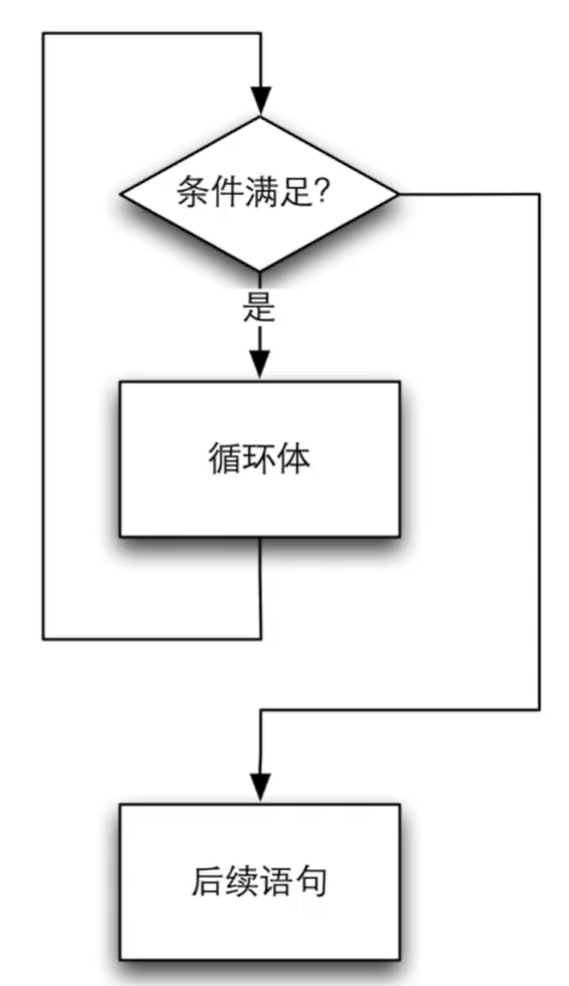Suzhou
• 阅读 1208

if语句可以判断条件是否满足，条件满足则执行相应动作；循环语句可以在满足条件时，不断地重复执行某些动作。 ::: tip 循环语句可以多次执行其他语句，它会检查一个逻辑条件是否满足，只在满足的时候执行它的循环体。 ::: while语句是一个循环语句，它会首先判断一个条件是否满足，如果条件满足，则执行后面紧跟着的语句或语句括号，然后再次判断条件是否满足，如果条件满足则再次执行，直到条件不满足为止。后面紧跟的语句或语句括号，就是循环体。

do-while循环和while循环很像，唯一的区别是我们在循环体执行结束的时候才来判断条件。也就是说，无论如何，循环都会执行至少一遍，然后再来判断条件。与while循环相同的是，条件满足时执行循环，条件不满足时结束循环。

## while循环

``````#include <stdio.h>

int main()

{
int n = 0;
int x;
printf("输入要判断的数字");
scanf("%d",&x);
n++;
x /= 10;
while(x > 0){
n++;
x /= 10;
}
printf("%d",n);
return 0;
} ``````

::: warning 上述程序最大支持查询10位数字的位数查询。 :::

::: tip

if（条件）{语句}当括号内条件满足时执行语句。while语句只需要将if语句的关键字换成while即可。 if语句中是一次性的，while是需要反复执行的。 ::: while循环流程图：::: tip 循环体内要有改变条件的机会，否则程序将一直在循环体中循环执行下去。 （防止语法正确逻辑错误的情况）。 ::: while循环的意思是：当条件满足时，不断重复执行循环体内的语句。 在进入while循环之前先判断条件，如果条件不满足，就不会进入循环体。

``````#include <stdio.h>

int main()

{
int n = 0;
int x;
printf("输入要判断的数字");
scanf("%d",&x);
while(x > 0){
n++;
x /= 10;
}
printf("%d",n);
return 0;
} ``````

``````#include <stdio.h>

int main()

{
int n = 0;
int x;
printf("输入要判断的数字");
scanf("%d",&x);
if (x > 0) {
while(x > 0){
n++;
x /= 10;
}
}else{
n=1;
}
printf("%d",n);
} ``````

``````#include <stdio.h>

int main()

{
int n = 0;
int x;
printf("输入要判断的数字");
scanf("%d",&x);
if (x > 0) {
while(x > 0){
n++;
x /= 10;
printf("n的值为%d x的值为%d\n",n,x);
}
}else{
n=1;
}
printf("%d",n);
} ``````
``````输入要判断的数字2541264
n的值为1 x的值为254126
n的值为2 x的值为25412
n的值为3 x的值为2541
n的值为4 x的值为254
n的值为5 x的值为25
n的值为6 x的值为2
n的值为7 x的值为0
7``````
``````输入要判断的数字4
n的值为1 x的值为0
1``````

``````#include <stdio.h>

int main()

{
int n = 0;
int x;
printf("输入要判断的数字");
scanf("%d",&x);
int hr = 0;
if (x > 0) {
while(x > 0){
hr++;
printf("hr%d\n",hr);
n++;
x /= 10;
printf("n的值为%d x的值为%d\n",n,x);
}
}else{
n=1;
}
printf("%d",n);
} ``````
``````输入要判断的数字952
hr1
n的值为1 x的值为95
hr2
n的值为2 x的值为9
hr3
n的值为3 x的值为0
3``````

::: tip 也可直接printf("hr\n")，起到观察程序运行位置的作用即可。 :::

## do while循环

``````#include <stdio.h>

int main()
{
int x;
int n = 0;
scanf("%d",&x);
do
{
x /= 10;
n++;
}while (x>0);
printf("%d",n);
return 0;
}``````

## for循环

``````#include <stdio.h>

int main()
{
int n;
scanf("%d",&n);
int fact = 1;
int i = 1;
while(i <= n){
fact *= i;
i++;
}
printf("%d!=%d\n",n,fact);
return 0;
}``````

``````#include <stdio.h>

int main()
{
int n;
scanf("%d",&n);
int fact = 1;
int i = 1;
for(i = 1; i <= n; i++){
fact *= i;
}
printf("%d!=%d\n",n,fact);
return 0;
}``````

``````for(i=0;i<5;i=i+1){
printf("%d,"i);
}``````

::: 上述程序中，循环控制变量i只在循环里面被使用，可以把变量i的定义写在for语句内，如下：

``````#include <stdio.h>

int main()
{
int n;
scanf("%d",&n);
int fact = 1;
//int i = 1;
for(int i = 1; i <= n; i++){
fact *= i;
}
printf("%d!=%d\n",n,fact);
return 0;
}``````

for可以认为是“对于”的意思，即对于（初始条件）...下，当（满足循环条件）...时，重复执行循环体，每一轮执行完毕时候，使得（循环动作）...。 ::: warning 做求和运算的程序时，记录结果的变量初始化应该为0，做求积的运算程序时，记录结果的变量应该初始化为1。 :::

``````#include <stdio.h>

int main()
{
int n;
scanf("%d",&n);
int fact = 1;
int i = 1;
for(i = 2; i <= n; i++){
fact *= i;
}
printf("%d!=%d\n",n,fact);
return 0;
}``````

``````#include <stdio.h>

int main()
{
int n;
scanf("%d",&n);
int fact = 1;
int i = 1;
for(i = n; i > 1; i--){
fact *= i;
}
printf("%d!=%d\n",n,fact);
return 0;
}``````

## 循环计算和选择

``````#include <stdio.h>

int main()
{
int i;
for(i=0;i<5;i++){
printf("i=%d ",i);
}
printf("\n最终i=%d",i);
return 0;
}``````
``````\$ cpp main.c -o main.ii
\$ cc main.ii -o main
\$ ./main
i=0 i=1 i=2 i=3 i=4

Program exited with status 0``````

``````#include <stdio.h>

int main()
{
int i;
for(i=1;i<=5;i++){
printf("i=%d ",i);
}
printf("\n最终i=%d",i);
return 0;
}``````
``````\$ cpp main.c -o main.ii
\$ cc main.ii -o main
\$ ./main
i=1 i=2 i=3 i=4 i=5

Program exited with status 0``````

``````     for(int i=1;i<=n;i++){
fact *= i;
}

int i = 1;
while(i <= n){
fact *= i;
i++;
}``````Suzhou
Lv1

3

0

0